# LAKHMIR SINGH AND MANJIT KAUR Solutions for Class 9 Physics Chapter 4 - Work and energy

Page / Exercise

## Chapter 4 - Work and energy 145

Solution 1

Mass = m

Height above the ground = h

Work done = Potential energy acquired by the body = m x g x h

where g is acceleration due to gravity

Solution 2

SI unit of work is Joule (J).

Solution 3

Work is a scalar quantity.

Work, as a physical quantity, requires only magnitude to be represented. Hence, it is scalar quantity.

Solution 4

When a force of 1 newton moves a body through a distance of 1 metre in its own direction, then the work done is known as 1 joule.

Solution 5

The condition for a force to do work on a body is that it should produce motion in the body.

Solution 6

Energy is a scalar quantity. It has only magnitude but no direction.

Solution 7

a) Unit of work is joule.

b) Unit of energy is joule.

Solution 8

The work done against gravity is zero when a body is moved horizontally along a frictionless surface because force of gravity acts perpendicular to the direction of motion.

Solution 9

Kinetic energy will become four times when the speed is doubled because kinetic energy is directly proportional to square of speed of the bodySolution 10

Mass = m

Velocity = v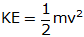Solution 11

Kinetic energy will become one-fourth when the speed is halved because kinetic energy is directly proportional to square of speed of the bodySolution 12

The kinetic energy of a body depends on

a) Mass of the body, m

b) Square of the velocity of the body, v2

Solution 13

Doubling the velocity would have a greater effect on kinetic energy.

Solution 14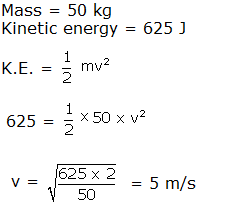Solution 15

a) Both kinetic and potential energy

b) Both kinetic and potential energy

c) Only kinetic energy

d) Only potential energy

e) Only potential energy

Note: In all the above cases we take ground as reference level where potential energy is zero.

Solution 16

Let masses of body A and B be m

Height of body A = h

Height of body B = 2h

Potential energy for body A, PEA = m x g x h

Potential energy for body B, PEB = m x g x 2h

Ratio = PEA : PEB ==Solution 17

Mass = 1 kg

Velocity = 2 m/s

K.E. === 2 J

Solution 18

Potential energy is a scalar quantity as it has magnitude only and it does not require any specification of direction.

Solution 19

Mass = 100 kg

Height = 5 m

g = 9.8 m/s2

P.E. = m x g x h = 100 x 5 x 9.8 = 4900 J

Work done is equal to PE acquired by the body.

Solution 20

False.

PE = m x g x h

= 1 x 9.8 x 1 = 9.8 J

Solution 21

The potential energy is doubled when the height is doubled since potential energy is directly proportional to height, h to which body is raised.

PEh

Solution 22

a) Potential energy

b) Kinetic energy

c) Potential energy

d) Potential energy

e) Potential energy

Solution 23

a)Force ; distance

b)Zero

c)newton; metre; force

d)Energy; kinetic energy

e)mechanical

Solution 24

The work done by a force on a body depends on two factors

a) magnitude of force

b) distance through which the body moves

Work done is directly proportional to the force applied and the distance through which the body moves.

W = F x s

where W is work done, F is force applied and s is distance through which the body moves.

Solution 25

Yes, it is possible that a force is acting on a body but still work done is zero. For example, in the case of a man pushing a wall, the work done is zero despite of non-zero force, since there is no displacement of the wall.

## Chapter 4 - Work and energy 146

Solution 26

a) Work done by force applied by the boy is positive because this force is in the direction of motion of the body.

b) Work done by the gravitational force is negative because this force is against the direction of motion of the body.

Solution 27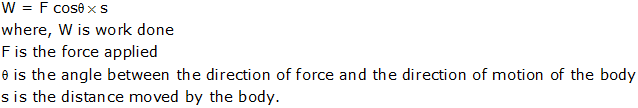Solution 28

Kinetic energy is directly proportional to the mass of the body, m.

KEm

Kinetic energy is directly proportional to square of speed of the body, v

KEv2

Solution 29

(a) Positive work: Work done by the force applied by a person on a ball that is thrown upwards.

(b) Negative work: Work done by gravitational force of earth on a ball thrown upwards.

(c) Zero work: Work done by gravitational force of earth on a box that is sliding horizontally on the ground.

Solution 30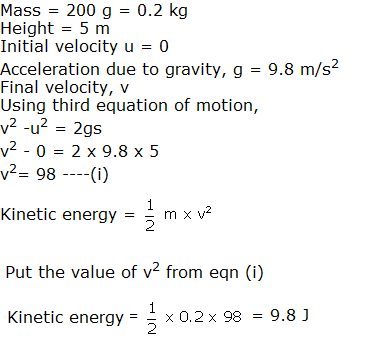Solution 31

KE = 20 J

Mass = 100 g = 0.1 kg

KE =20 =v2 = 400

v = 20 m/s

Momentum = m x v = 0.1 x 20 = 2 kg.m/s

Solution 32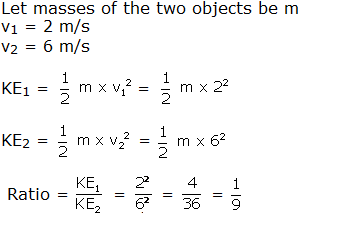Solution 33

Mass of body = 2 kg

Initial velocity u = 0

Time taken = 2 s

Acceleration due to gravity, g = 10 m/s2

Final velocity v

Using first equation of motion

v = u + gt

= 0 + 10 x 2 = 20 m/s

KE === 400 J

Solution 34

Mass of scooter+scooterist = 150 kg

Initial velocity u = 10 m/s

Final velocity v = 5 m/s

Retardation = a

Distance covered= s

Using third equation of motion

v2 - u2 = 2as

52 - 102 = 2as

as = -75/2 ------(i)

Work done W = F x s

But F = m x a

So, W= m x a x s

Put the value of 'as' from eq(i)

W = 150 x (-75/2) = -5625 J

Neagtive sign implies that force of brakes acts opposite to the direction of motion.

Solution 35

Mass of rock = 10 kg

Height of ladder, h = 5 m

Initial velocity of rock, u = 0

Final velocity v

g = 10 m/s2

using third equation of motion

v2 - u2 = 2gh

v2 - 02 = 2 x 10 x 5

v = 10 m/s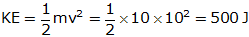Solution 36

Mass of car = 1000 kg

Initial velocity u = 20 m/s

Final velocity v = 10 m/s

Retardation = a

Distance covered = s

Using third equation of motion

v2 -u2 = 2as

102- 202 = 2as

as = -150 ------(i)

Work done W = F x s

But F = m x a

So, W= m x a x s

Put the value of 'as' from equation (i)

W = 1000 x -150 = -150000 = -150 kJ
Neagtive sign implies that force of brakes acts opposite to the direction of motion.

Solution 37

Mass of body, m = 100 kg

Height , h= 10 m

Acceleration due to gravity, g = 10 m/s2

i) Work done, W = m x g x h = 100 x 10 x 10 = 10000 = 10 kJ

ii) Potential energy of the body = work done = 10 kJ

Solution 38

Mass of the boy, m = 50 kg

Height, h = 100 m

Acceleration due to gravity, g = 9.8m/s2

Work done by the boy, W = m x g x h = 50 x 9.8 x 100 = 49000 J = 49 kJ

Potential energy gained by the boy = work done by the boy = 49 kJ

Solution 39

Work done by a force applied on a body is

i) positive when the force acts in the direction of motion of the body.

ii) negative when the force acts in the direction opposite to the direction of motion of the body.

iii) zero when the force acts at right angle to the direction of motion of the body.

Solution 40

Mass of the box, m = 150 kg

PE = 7350 J

Acceleration due to gravity, g = 9.8m/s2

PE = m x g x h

7350 = 150 x 9.8 x h

h== 5 m

Solution 41

Mass of the body, m = 2 kg

Initial velocity, u = 20 m/s

Acceleration due to gravity, g = 10 m/s2

Height reached = h

Time, t = 2 s

Using second equation of motion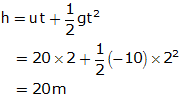PE after 2 s = m x g x h = 2 x 10 x 20 = 400 J

Solution 42

Force, F = 1 N

Distance, s = 1 m

Work done W = F x s

= 1 x 1 = 1 J

Solution 43

Force, F = 2.5 x 1010 N

Velocity, v = 5 m/s

Time, t = 2 minutes = 120 s

Distance, s = v x t = 5 x 120 = 600 m

Work done, W = F x s = 2.5 x 1010 x 600 = 15 x 1012 J

Solution 44

A stretched rubber band is an example of a body possessing energy while it is not in motion. The rubber band contains potential energy due to the change in its shape or configuration.

Solution 45

a) Gravitational potential energy of a body depends on:

i) mass of the body, m

ii) height to which the body is lifted, h

iii) acceleration due to gravity, g

b)

i. A moving cricket ball has kinetic energy

ii. A stretched rubber band has potential energy

Solution 46

Two examples where a body possesses both kinetic energy as well as potential energy are

i) a man climbing up a hill

ii) a flying aeroplane

Solution 47

Mass of man, m

Height of tree, h = 5 m

Work done, W = 2500 J

Acceleration due to gravity, g = 10 m/s2

W = m x g x h

2500 = m x 10 x 5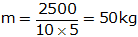Solution 48

Work done, W =24.2 J

Distance, s = 20 cm = 0.2 m

Force, F

W = F x s

24.2 = F x 0.2

F = 24.2/0.2 = 121 N

Solution 49

Mass of boy, m = 40 kg

Height, h = 1.5 m

Acceleration due to gravity, g = 10 m/s2

i) At highest point, velocity, v = 0

Therefore KE = 0

ii) PE = m x g x h = 40 x 10 x 1.5 = 600 J

Solution 50

a) Potential energy

b) Both potential and kinetic energy

## Chapter 4 - Work and energy 147

Solution 51

Mass, m = 200 kg

Height, h = 2 m

Acceleration due to gravity, g = 9.8 m/s2

i) Potential energy = m x g x h = 200 x 9.8 x 2 = 3920 J

ii) Work done is against gravity = Potential energy gained by the weights, therefore

Work done W = m x g x h = 200 x 9.8 x 2 = 3920 J

Solution 52

(a) Work is done when a force applied on a body produces motion in it.

Formula for work done:

W = F x s

where W is the work done

F is force applied

S is the displacement of the body in the direction of force

(b) Mass of the person, m = 50 kg

Height of tower, h = 72 m

Acceleration due to gravity = 9.8 m/s2

Work done W = m x g x h

= 50 x 9.8 x 72 = 35280 J

Solution 53

(a) Work is said to be done when the force applied on a body produces motion in it.

Work done by a body in moving up is given by

W = m x g x h

where W is the work done against the gravity

m = mass of the body

g = acceleration due to gravity

h = height through which the body is lifted above the ground

(b) Force, F = 2 N

Distance, s = 10 cm = 0.1 m

Work done W = F x s = 2 x 0.1 = 0.2 J

Solution 54

(a) When the displacement of a body is at right angles to the direction of foce acting on it, then work done is zero.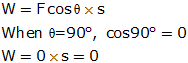(b) Force, F = 50 N

Distance, s = 4 m

Angle between direction of force and direction of motion,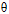= 60o

Work done, W = F cosx s

= 50 x cos60o x 4 = 50 x 0.5 x 4= 100 J

Solution 55

(a) Energy is the ability to do work. SI unit of energy is Joule.

(b) Various forms of energy are:

1. Kinetic energy

2. Potential energy

3. Chemical energy

4. Heat energy

5. Light energy

6. Sound energy

7. Electrical energy

8. Nuclear energy

(c) Let masses of bodies be m

Velocity of one body, v1= v

Velocity of another body, v2 = 2v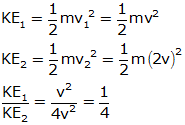Solution 56

(a) The energy of a body due to its motion is called kinetic energy

(b) When the velocity becomes zero, the kinetic energy also becomes zero since kinetic energy is directly proportional to square of the velocity.

(c)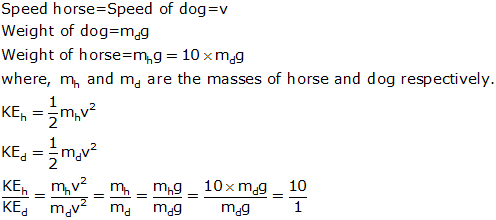Solution 57

(a) The energy of a body due to its position or change in its shape is known as its potential energy. E.g. a stretched rubber has potential energy due to change in its shape and water in the overhead tank has potential energy due to its height above the ground.

PE = m x g x h

where, PE is the potential energy of the body,

m is the mass of the body,

g is the acceleration due to gravity,

h is the height above the surface ofearth.

(b) Kinetic energy of a body is due to motion of the body while potential energy is due to position or change in shape of the body.

Kinetic energy is zero for a still body, while potential energy may or may not be zero for a still body.

Kinetic energy of a body is directly proportional to its speed while potential energy is directly proportional to the height to which the body is above the ground.

(c) Mass of ball, m = 0.5 kg

Speed v1 = 5 m/s

Speed v2 = 3 m/s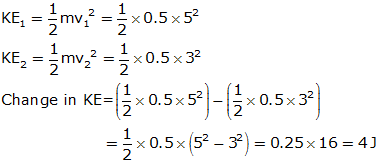Solution 58

(a) The potential energy due to the position of the body above the ground is gravitational potential energy and the potential energy due to change in shape and size of the body is elastic potential energy. E.g. a stretched rubber has elastic potential energy due to change in its shape while water in the overhead tank has gravitational potential energy due to its height above the ground.

(b) Work done, W = 784 J

Mass, m = 20 kg

g = 9.8 m/s2

W = m x g x h

784 = 20 x 9.8 x h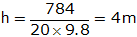## Chapter 4 - Work and energy 148

Solution 71

Work done is more on the bicycle because the truck does not move at all and the bicycle moves through a certain distance. And work is said to be done only when applied force produces motion in the body.

## Chapter 4 - Work and energy 149

Solution 72

The work done will decrease as the angle between the direction of force and direction of motion is increased gradually because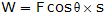And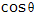decreases as the angleis increased.

Solution 73

The work done will be zero when angle between the direction of force and direction of motion is 90o because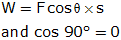Solution 74

Work done will be maximum when angle between the direction of force and direction of motion is 0o because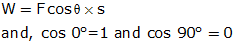Solution 75

The work done is zero because the gravitational force acts along the radius of the circular path, at right angles i.e. 90o to the motion of satellite.

Solution 76

Weight of man = Mg = 800 N

Weight of package = mg = 200 N

Total weight of man and package = Mg + mg = (M+m)g = 1000 N

Height of the summit, h = 1200 m

i) Work done = (M+m) x g x h = 1000 x 1200 = 12 x 105 J

ii) Potential energy of the package = m x g x h = 200 x 1200 = 2.4 x 105 J

Solution 78

The work done by both X and Y are equal because irrespective of whether they reach the top of building by using a spiral or slanted ladder, the vertical distance moved by them against the gravity is same.

Solution 79

Yes, the kinetic energy of the ball thrown inside a moving bus depends on the speed of the bus because the speed of the bus adds up to the speed with which the ball is thrown inside the moving bus.

Solution 80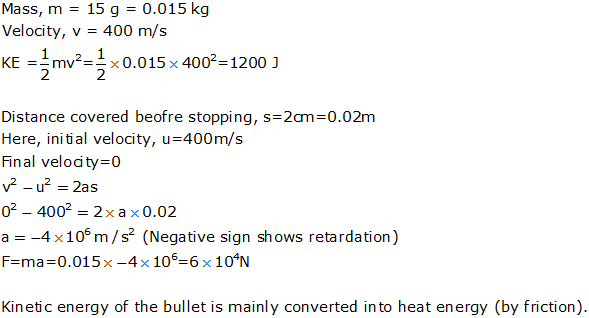Solution 77
Potential energy becomes maximum.

## Chapter 4 - Work and energy 162

Solution 1

The commercial unit of energy is kilowatt-hour.

Solution 2

One kilowatt-hour is the amount of electrical energy consumed when an electrical appliance having power of 1 kilowatt is used for 1 hour.

Solution 3

Megawatt and kilowatt are the units of power bigger than watt.

Solution 4

1 watt is the power of an appliance which does work at the rate of 1 joule per second.

Solution 5

1 horse power = 746 watt

Solution 6

Power has watt as its physical unit

Solution 7

1 watt

Solution 8

Work done = 1200 J

Time taken = 2 minutes = 2 x 60 = 120 s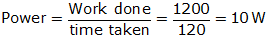Solution 9

One kilowatt = 3.6 x 106 J

Solution 10

a) Power

b) Electrical Energy

Solution 11

1 kW-h of electrical energy is commonly known as unit of electricity.

Solution 12

A cell converts chemical energy into electrical energy.

Solution 13

Electric motor

Solution 14

a) Electric generator

b) Cell

c) Electric iron

d) Solar cell

e) Electric bulb

Solution 15

a) Speaker

b) Steam engine

c) Car engine

d) Gas stove

e) Solar water heater

Solution 16

a) Work

b) Joule ; second

c) kWh

d) conservation; transformed; created; destroyed

e) kinetic; potential

## Chapter 4 - Work and energy 163

Solution 17

Force, F = 400 N

Distance, s = 60 m

Time taken, t = 1 minute = 60 s

Work done, W = F x s = 400 x 60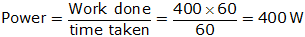Solution 18

At a hydroelectric power station, the potential energy of water is transformed into kinetic energy and then into electrical energy.

Solution 19

At a coal-based thermal power station, the chemical energy of coal is transformed into heat energy, whhich is further converted into kinetic energy and electrical energy.

Solution 20

Weight of the man = 500 N

Weight of the load = 100 N

Total weight = 600 N

Height of stairs = 4 m

Time taken = 5 s

Work done = mg x h = weight X h = 600 x 4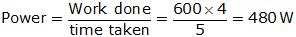Solution 21

Power = 3 kW

Time = 20 s

Power =Work done = power x time = 3 x 20 kWs = 60 kJ

Solution 22

Energy consumed = 600 kJ

Time taken = 5 minutes = 300 s

Power === 2 kW

Solution 23

Power = 100 W

a)time = 1 s

energy = power x time = 100 J

b)time = 1 minute = 60 s

energy = power x time= 100 x 60 = 6 kJ

Solution 24

Power of 1 fan = 120 W

Power of 5 fans = 5 x 120 = 600 W = 0.6 kW

Time = 4 hours

Electrical energy = 0.6 x 4 = 2.4 kWh

Solution 25

A radio first converts electrical energy into kinetic energy and then into sound energy

Solution 26

In an electric bulb, electrical energy is first converted into heat energy and then into light energy

Solution 27

Fan, washing machine, mixer grinder, water pump, hair dryer use electric motor

Solution 28

i) chemical energy to electrical energy

ii) electrical energy to heat and light energy

Solution 29

i) Maximum potential energy is present in the bob at point C

as at point C bob is at maximum height.

ii) Maximum kinetic energy is present in the bob at point A as at point A bob is at maximum speed

Solution 30

Weight of the car = 20000 N= 20 kN

Speed = 8 m/s

Distance s= 120 m

Time = 100 s

a) Work done W = f x s = 20 x 120 = 2400 kJ

b) Power === 24 kW

Solution 31

a) The change of one form of energy into another form of energy is known as transformation of energy, e.g. in a cell chemical energy is transformed into electrical energy

b)

a. When a ball is thrown upwards its kinetic energy gradually converts into potential energy and potential energy becomes maximum at the maximum height attained by the ball

b. When a stone is dropped from the roof of the building its potential energy gradually converts into kinetic energy and kinetic energy becomes maximum when the stone is just above the ground

Solution 32

a)Law of conservation of energy states that whenever energy changes from one form to another form, the total amount of energy remains constant. Energy can never be created nor destroyed, it transforms from one form to another. For example, when electrical energy is converted into light energy in an electric bulb, then some energy is wasted as heat during conversion but the total energy remains the same.

b)Initially the pendulum is at rest. The bob is pulled to one side to position B to give it potential energy due to higher position of B and then released, the bob starts swinging

i.When the bob is at position B, it has potential energy but no kinetic energy

ii.As the bob starts moving down from position B to A, its potential energy starts decreasing and kinetic energy starts increasing

iii.When the bob is at position A, it has maximum kinetic energy and zero potential energy

iv.As the bob starts moving down from position A to C, its kinetic energy starts decreasing and potential energy starts increasing

v.On reaching the extreme position C, the bob stops for a very small instant of time and bob maximum potential energy and zero kinetic energy.

Therefore at extreme positions B and C bob has only potential energy and at A it has only kinetic energy and at other intermediate positions bob has both kinetic and potential energy. Thus the total energy of the pendulum is same for any instant of time (conserved)Solution 33

a) The unit kWh stands for kilowatt-hour. One kilowatt-hour is the amount of electrical energy consumed when an electrical appliance having power of 1 kilowatt is used for 1 hour. It represents the amount of electrical energy consumed in 1 hour.

b) Power = 1000 W = 1 kW

Time = 60 minutes = 1 hour

Energy(kWh) = 1 x 1 = 1 kWh

Solution 34

a) 1 kilowatt-hour = 1 kW for 1 hour

= 1000 W for 1 hour

But 1 watt =1 kilowatt- hour =for 1 hour

=for 3600 seconds

= 1000 x 3600 = 3.6 x 106 joule

b) Energy consumption = 650 units = 650 kWh

1 kWh = 3.6 x 106 J

650 kWh = 3.6 x 650 x 106 =2.34 x 109 J

Solution 35

a) Power is the rate of doing work.

Power =SI unit of power is watt(W)

b) Mass of the boy = 40 kg

Mass of the box = 20 kg

Total mass = 60 kg

Height h= 15 m

g = 10 m/s2

time taken = 25 s

work done = m x g x h = 60 x 15 x 10 = 9000 J

Power === 360 W

## Chapter 4 - Work and energy 164

Solution 46

Mass of body = 1 kg

g = 10 m/s2

PE = mgh = 1 x 10 x h = 10 x h

KE ===i. At h = 5 m; v = 0 m/s

PE = 10 x 5 = 50 J

KE = 0 J

Total energy = PE + KE = 50 J

ii. At h = 3.2 m; v = 6 m/s

PE = 10 x 3.2 = 32 J

KE == 18 J

Total energy = PE + KE = 32+ 18 = 50 J

iii. At h = 0 m; v = 10 m/s

PE = 0 J

KE == 50 J

Total energy = PE + KE = 50 J

The total energy in all three cases is constant. This proves the law of conservation of energy

Solution

## Chapter 4 - Work and energy 165

Solution 48

No. of steps = 28

Height of each step = 28 cm

Total height = 20 x 28 = 560 cm = 5.6 m

Mass of student = 55 kg

g = 9.8 m/s2

time = 5.4 s

Work done = m x g x h = 55 x 9.8 x 5.6 = 3018.4 J

Power === 559 W

Solution 49

Weight of box = 100 N

Height = 1.5 m

i. work done = m x g x h = 100 x 1.5 = 150 J

ii. potential energy = m x g x h= 100 x 1.5 = 150 J

iii. weight of 4 boxes = 400 N

time = 1 minute = 60 s

work done = 400 x 1.5 = 600 J

power === 10 W

Solution 50

a) Electrical energy to sound energy

b) Sound energy to electrical energy

c) Electrical energy to light (and heat) energy

d) Chemical energy to electrical energy to light energy(and heat energy)

### STUDY RESOURCES

REGISTERED OFFICE : First Floor, Empire Complex, 414 Senapati Bapat Marg, Lower Parel, Mumbai - 400013, Maharashtra India.